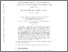# Exit problems related to the persistence of solitons for the Korteweg-de Vries equation with small noise

De Bouard, Anne and Gautier, Eric (2010) Exit problems related to the persistence of solitons for the Korteweg-de Vries equation with small noise. Discrete and Continuous Dynamical Systems, 26. pp. 857-871.Preview
Text
Official URL: http://tse-fr.eu/pub/30198

## Abstract

We consider two exit problems for the Korteweg-de Vries equation perturbed by an additive white in time and colored in space noise of amplitude [epsilon] . The initial datum gives rise to a soliton when [epsilon=0] . It has been proved recently that the solution remains in a neighborhood of a randomly modulated soliton for times at least of the order of [epsilon-2] . We prove exponential upper and lower bounds for the small noise limit of the probability that the exit time from a neighborhood of this randomly modulated soliton is less than [T] , of the same order in [epsilon] and [T] . We obtain that the time scale is exactly the right one. We also study the similar probability for the exit from a neighborhood of the deterministic soliton solution. We are able to quantify the gain of eliminating the secular modes to better describe the persistence of the soliton.

Item Type: Article English 2010 Yes B- ECONOMIE ET FINANCE TSE-R (Toulouse) UT1 18 Apr 2016 14:06 07 Mar 2018 13:23 oai:tse-fr.eu:30198 http://publications.ut-capitole.fr/id/eprint/19704

### Actions (login required)View Item